DAX as a Query Language Part 2 - Excelerator BI

# DAX as a Query Language Part 2

Level: Intermediate

In my last article I introduced DAX as a Query Language and explained why learning to query your data model can be useful.  I covered the basics about using a tool like DAX Studio to query a database and return a table of data as a result.  In this article I am going to go deeper and introduce the more common and useful query functions including CROSSJOIN, SUMMARIZE and ADDCOLUMNS (there are more functions that I don’t cover in this article).Here is the link to the PBIX data model again if you want to follow along and do the examples yourself.  I am using DAX Studio again to connect to Power BI Desktop running on my location machine.  If you take the time to complete these examples you will significantly increase your learning and knowledge retention.

## Lineage

Before I move on, an interesting and important fact about Power Pivot (when compared to traditional database tools) is the concept of lineage (pronounced LIN-E-AGE).  When a new virtual table is created in a query or a formula in Power Pivot, the new table will include an automatic one to many relationship to the table from where it was created.  Consider the following simple table from last time.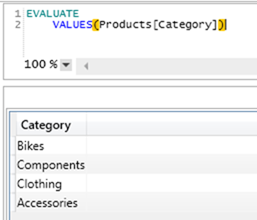You can see above that this query produces a single column table of all of the unique product categories.  This new virtual table retains lineage to the data model.  In this case the new virtual table comes from the Products table and hence the new virtual table has lineage to the Products table.  You can “imagine” this as shown below with the new table having a 1 to many relationship to the Products table.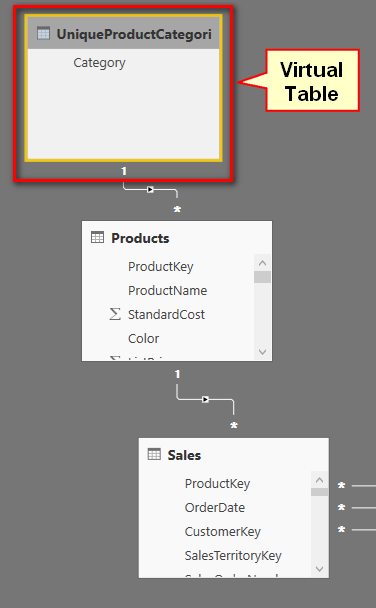Note the image above is just a visual simulation of what happens.  The virtual table is not materialised and you cannot actually see it in the relationship view. But the virtual table does exist (virtually) and the lineage to the Products table also exists – it is just that you can’t actually see it.  I recommend that you learn to “imagine” this happening in the data model in your mind as it will help you understand how the new virtual table interacts with the rest of the data model especially as it relates to context transition.

All virtual tables have lineage to the tables from where they came from.

## CROSSJOIN

CROSSJOIN is a function that can create a new table from 2 or more source tables. For this example I am going to join some virtual tables. The first virtual table is VALUES(Product[Category]) which of course returns a list of all the unique product categories.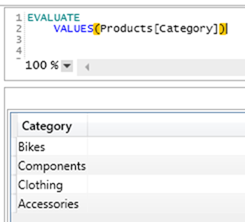The second virtual table is a list of all possible Customer gendersNext I am going to use CROSSJOIN to create a new table containing all the unique combinations of both tables.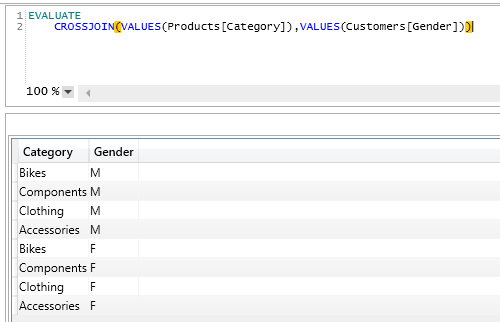In the table above there are 4 rows x 2 rows giving a total of 8 rows of all of the unique values.  Continuing on the concept of “imagining” the way these new virtual tables are related in the data model, it would look something like this: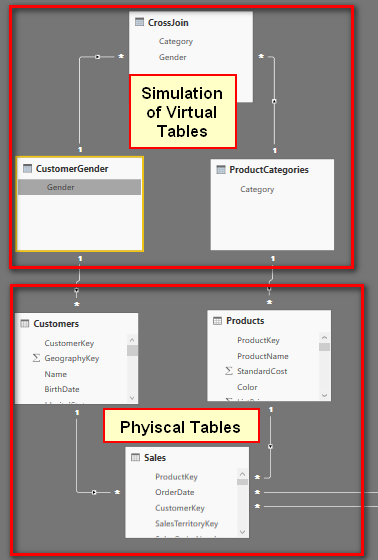Remember this is just a simulation of what it looks like.  These tables at the top are not materialised and you cannot see them in the data model.  But you can “imagine” them as looking like this and they behave in exactly the same way as they would if they were physical tables.

### M x N Can Mean a Large Table

You need to be careful with CROSSJOIN as by definition the resulting table will be m x n rows long where m is the number of rows in table 1 and n is the number of rows in table 2.  If I were to CROSSJOIN the customer table (18,484 rows) with the Products table (397 rows) I would end up with more than 7 million rows.  This in itself is not a problem for Power Pivot to create such a large table in memory, but it can definitely be a problem if you try to materialise the table.  More on that next week.## SUMMARIZE

SUMMARIZE is by far my favourite DAX Query function.  SUMMARIZE can do similar things to CROSSJOIN however CROSSJOIN can join tables that do not have relationships whereas SUMMARIZE can only join tables that are related with a many to 1 relationship.

SUMMARIZE first takes a table and then one or more columns (that can be reached via a many to 1 relationship) that you want to include in the new summarised table.

SUMMARIZE(<table>, table[column], table2[column],….)

Here is an example.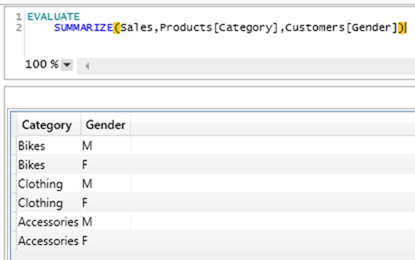The query results above are similar to the CROSSJOIN query from before but there is one important difference.    SUMMARIZE will only return rows that actually exist in the data itself (Note there are only 6 rows above compared with 8 rows in the CROSSJOIN example).

Consider the relevant tables from the data model below.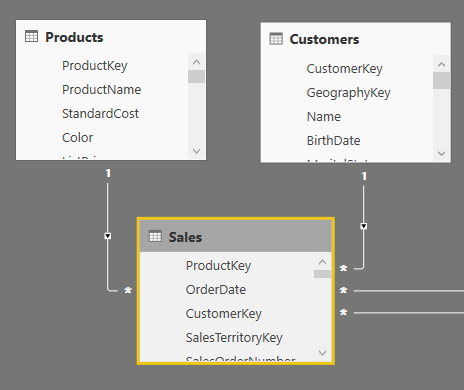Here is the SUMMARIZE formula written earlier.

```EVALUATE
SUMMARIZE(Sales, Products[Category], Customers[Gender])```

This query starts with the Sales table and then adds the Products[Category] column from the Products table and the Customers[Gender] column from the Customers table.  The 2 columns specified inside the SUMMARIZE formula come from tables on the 1 side of the many to 1 relationships – this is allowed.

The following is not allowed and will not work.

EVALUATE
SUMMARIZE(Products, Sales[CustomerKey])

It doesn’t work because the column Sales[CustomerKey] cannot be reached from the Products table via a many to 1 relationship.

It is also possible to write a SUMMARIZE statement over any single table.  In the example below, the SUMMARIZE statement returns a list of all possible combinations of product category and colour.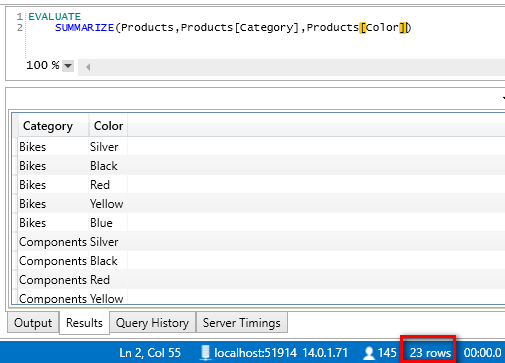You could also achieve the same result with the ALL function (which would be an easier solution if you are just using a single table)

```EVALUATE
ALL(Products[Category], Customers[Gender])```

### Adding Summary Sales to the Summary Table

So far the SUMMARIZE queries above are just lists of valid combinations.  It is time to do something more interesting and add the [Total Sales] to these summary tables.  Before moving on please note that the following formulas are not best practice – there is a better way which I will cover later.

Consider the following formula

```EVALUATE
SUMMARIZE(
Products,
Products[Category],
Products[Color],
"total sales", [Total Sales]
)```

Note specifically that the table parameter in this formula is “Products”.  Also note below that this formula returns blank rows (shown below).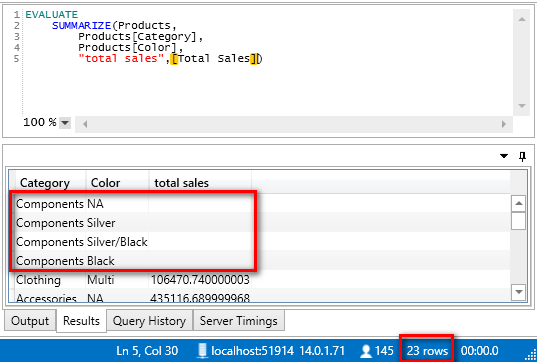This summarize statement correctly summarises all the combinations of Product[Category] and Product[Color] in the products table and then for those products where there are sales, those sales are shown next to the unique combination.  But in some cases the unique combination doesn’t actually have any sales, hence the blank rows.

### Using Sales as the Table Parameter

If I change the formula above and swap out the Products table with the Sales table, then the blank rows are no longer visible (see below).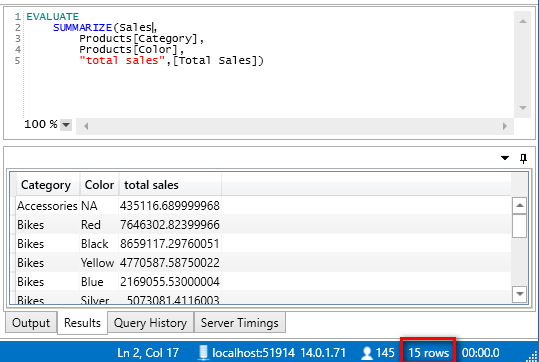SUMMARIZE will always find the unique combinations that actually exist in the selected data.  Because this new formula starts from the Sales table, only combinations of Product[Category] and Product[Color] where there are actual sales are returned.

### Context Transition or No Context Transition?

Those of you that are familiar with the concept of context transition may be thinking that context transition is occurring here.  That is a valid thing to assume but this is not what is happening here.  Consider the following formula.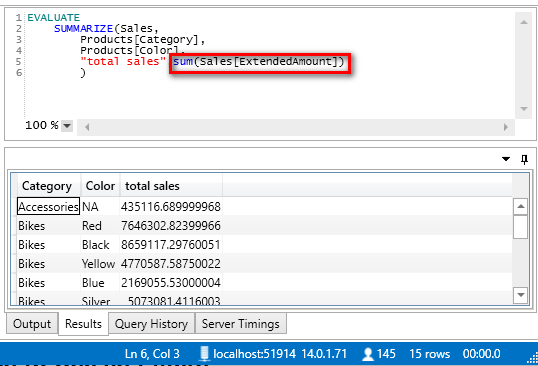Note how I have swapped out the measure [Total Sales] with SUM(Sales[ExtendedAmount]).  With this new formula above there is no CALCULATE forcing context transition yet despite this the table still returns the same result.  This implies that SUMMARIZE does not operate in a Row Context.  In fact SUMMARIZE is a Vertipaq Storage Engine operation.  The part that produces the valid combinations of columns is very efficient however the calculation of the total sales figures are very inefficient.  For this reason it is better to use ADDCOLUMNS to add the sales totals (see below).ADDCOLUMNS does exactly what it suggests – it adds new columns to a table in a query.  The general syntax is as follows:

To demonstrate how this works, let me start with a formula from earlier that produces the following table.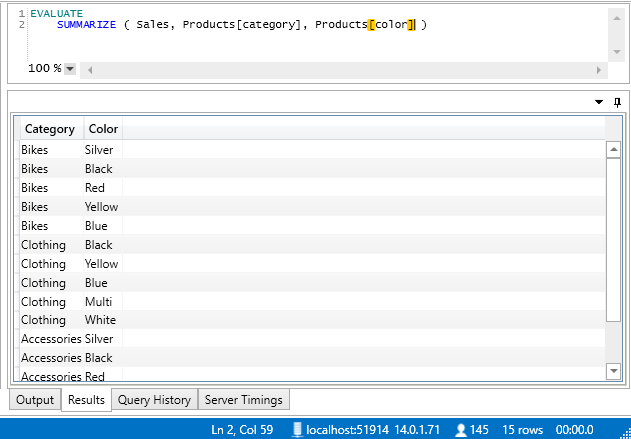The SUMMARIZE function returns a 2 column table with 15 rows – all the possible combinations that contains sales values.  This table can be used as the table parameter in the ADDCOLUMNS formula as follows.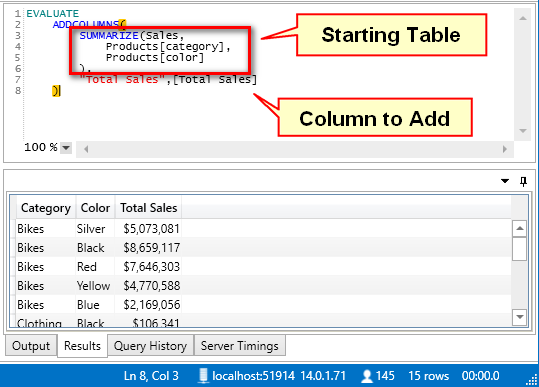You can see above that this new table returns the Total Sales for each of the 15 possible combinations.

And it is possible to add as many new columns as you need to the summary table.  See below.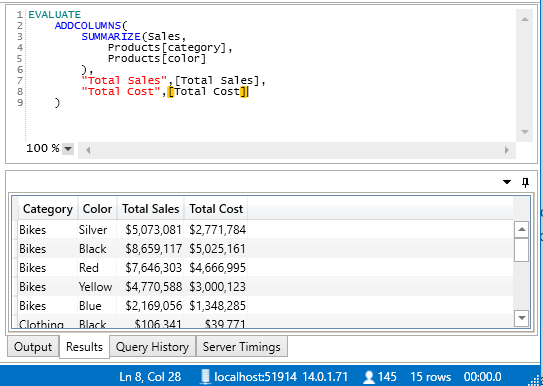### The Important Differences between ADDCOLUMNS and SUMMARIZE

Now you have seen that it is possible to add columns (such as summary total sales) to a table using SUMMARIZE and also with ADDCOLUMNS.  But there are some important differences between these 2 approaches.

#### ADDCOLUMNS has a Row Context

Unlike I showed with SUMMARIZE earlier in this article, ADDCOLUMNS does have a row context.  Consider the following query.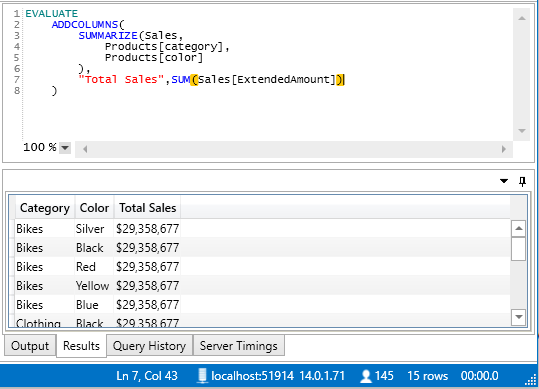When I swap out the measure [Total Sales] with SUM(Sales[ExtendedAmount]) the results are wrong.  This shows that ADDCOLUMNS operates in a row context.

#### Efficiency

When given the choice, you should choose to use ADDCOLUMNS in favour of SUMMARIZE to add these additional columns of data.  ADDCOLUMNS is a lot more efficient in the way it adds the values to the SUMMARIZE table. SUMMARIZE uses a Vertipaq Storage Engine operation to produce the base table and then ADDCOLUMNS leverages lineage and context transition to add the value columns – this approach leverages the special capabilities of Power Pivot to do the job in the most efficient way.  For a more detailed coverage of this topic you should read this article from The Italians.

## Other DAX Query Functions

I realise I have not covered all DAX Query functions in this series of articles.  There are others, some of which are only available in the newer versions of Power Pivot (eg Power BI Desktop, Excel 2016).  If you are interested to find out more you can do some research online.  I will be covering 1 final function next week – the ROW function.

## Uses for DAX Queries

Next week I will share my final article in this series where I explain a few ways that you can use DAX Queries in the real world.  Be sure to check back next week, or better still sign up to my weekly newsletter to be notified when there are new articles.

### 5 thoughts on “DAX as a Query Language Part 2”

1.2.Nice blog, Matt. A clear description of the DAX functions, and what works where and how.
Thanks

3.The concept behind SUMMARIZE together wit CROSSJOIN is the epitome of a Pivot Table and gives rise to key terms like auto-exists and many useful applications.

Great blog post Matt!

4.Excellent as usual 🙂 Thanks Matt !
Summarise is also one of my favourite DAX Function.
Just a question: how do you choose between DAX query functions and Power Query ?
Most of the time, both are possible. But I know Power Query uses ‘Query Folding’ engine and thus optimises the query performance.
For example, I saw some people using CrossJoin to create a Bridge Table and cope with M2M Relationships whereas I tend to use Power Query for this kind of task. What do you think ?

1.My personal preference is to push reusable concepts to the source (e.g. SQL Server). If they are not reusable I generally prefer to put them in Power Query particularly if they are heavy on transformation effort. But if you need to use a measure as part of the summary table, then clearly Power Pivot will be better. Personally I would always put a cross join that I wanted to materialise into Power Query, and I wouldn’t use crossjoin in this example unless the source data had all possible combinations

Scroll to Top